## 第十四章——循环神经网络（Recurrent Neural Networks）（第二部分）

RNN是一种可以预测未来（在某种程度上）的神经网络，可以用来分析时间序列数据（比如分析股价，预测买入点和卖出点）。在自动驾驶中，可以预测路线来避免事故。更一般的，它可以任意序列长度作为输入，而不是我们之前模型使用的固定序列长度。例如RNN可以将句子、文档、语音作为输入，进行自动翻译、情感分析、语音转文字。此外，RNN还用于作曲（谷歌Magenta项目作出的the one）、作文图片自动生成标题

## 14.1 周期神经元（Recurrent Neurons）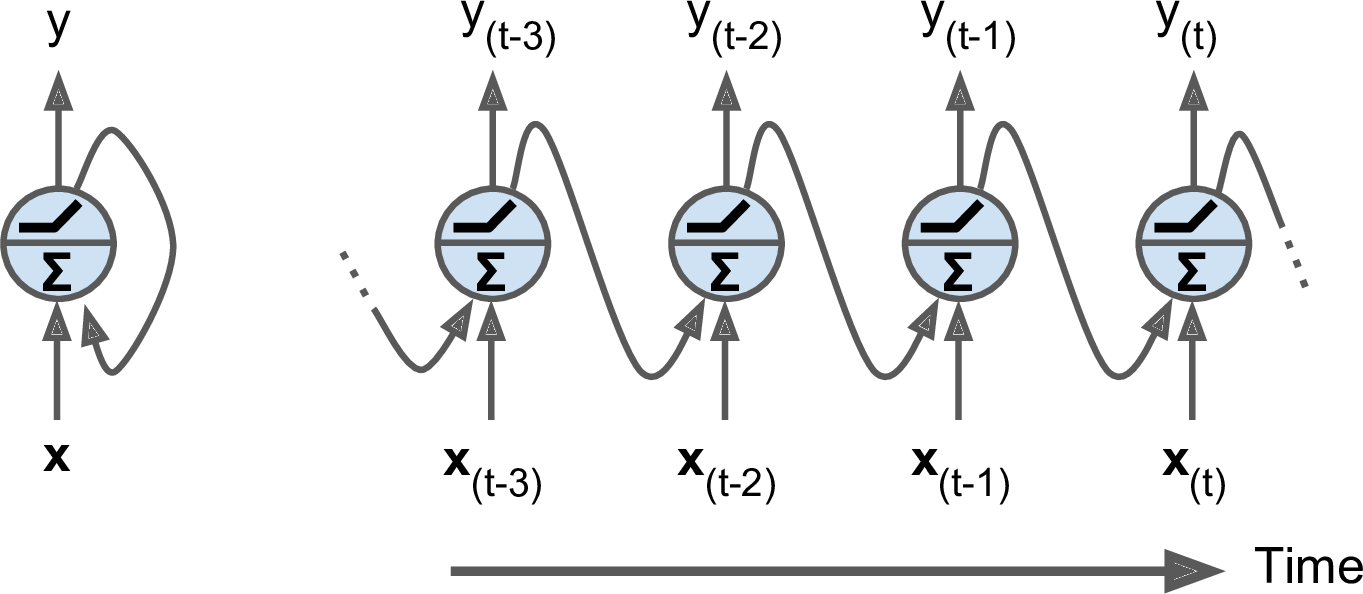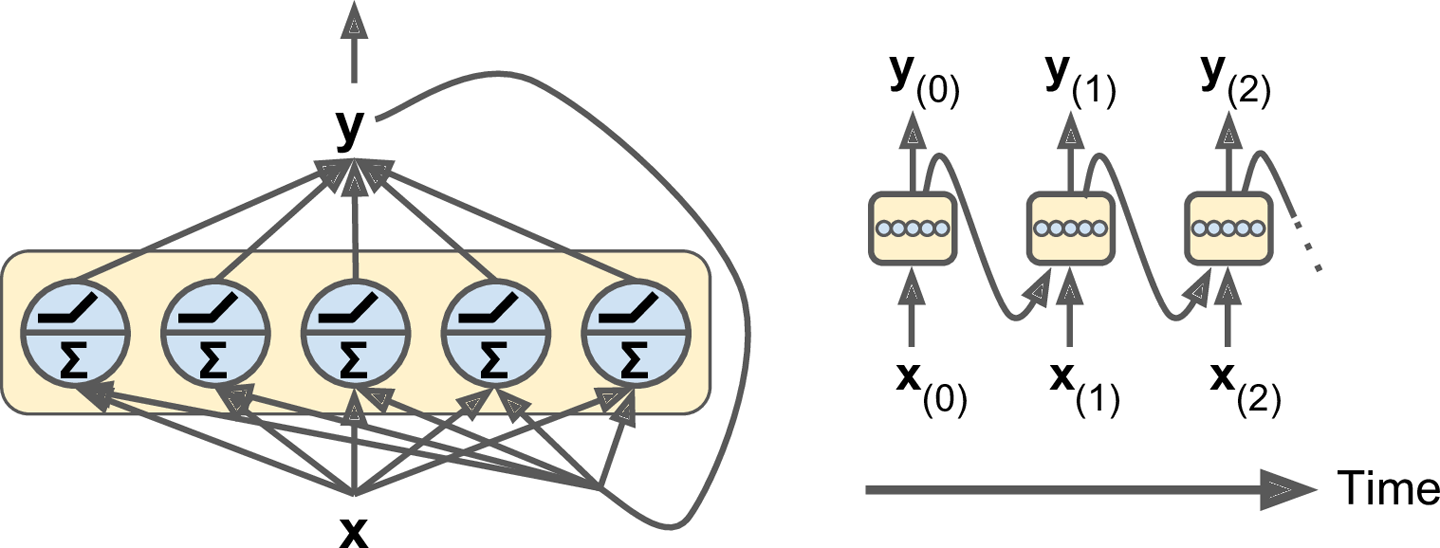\begin{align*}
y_{(t)} = \phi(x_{(t)}^T \cdot w_x + y_{(t-1)}^T \cdot w_y + b)
\end{align*}

\begin{align*}
Y_{(t)} &= \phi(X_{(t)}^T \cdot W_x + Y_{(t-1)}^T \cdot W_y + \textbf{b}) \\
&= \phi([X_{(t)} \quad Y_{(t)}] \cdot W + \textbf{b}) \quad \mbox{with} \quad
W = \begin{bmatrix}
W_x \\
W_y
\end{bmatrix}
\end{align*}

• $Y_{(t)}$是一个$m \times n_{\mbox{neurons}}$矩阵，包含该层在time step $t$关于整个mini-batch实例的输出（$m$是mini-batch的实例数，$n_{\mbox{neurons}}$是神经元数量）。
• $X_{(t)}$是一个$m \times n_{\mbox{inputs}}$矩阵，包含该time step $t$所有实例的输入（$n_{\mbox{inputs}}$是特征数）。
• $W_x$是一个$n_{\mbox{inputs}} \times n_{\mbox{neurons}}$矩阵，包含当前time step输入到输出的连接权重。
• $W_y$是一个$n_{\mbox{neurons}} \times n_{\mbox{neurons}}$矩阵，包含上个time step输出到当前time step输出的连接权重。
• $W$的形状是$(n_{\mbox{inputs}} + n_{\mbox{neurons}}) \times n_{\mbox{neurons}}$
• $\textbf{b}$是一个大小为$n_{\mbox{neurons}}$的向量，包含所有神经元的偏置项。

### 14.1.1 Memory Cells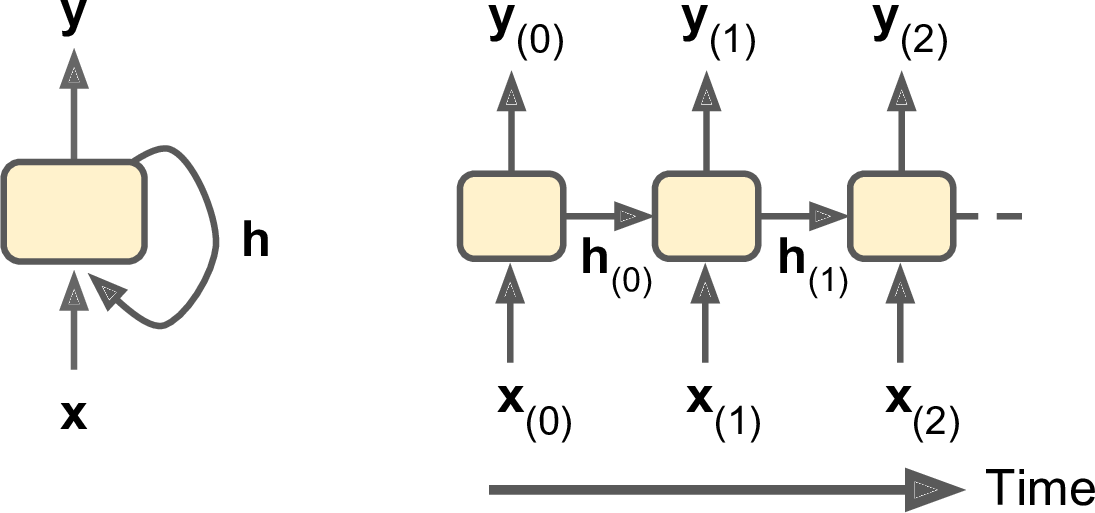### 14.1.2 输入和输出序列

RNN可以一个序列作为输入，再同时输出一个序列（如图14-4左上）。该模型可用于股价预测，输入前$N$天的股价，输出每一天的股价，知道第$N+1$天。每增加一天的输入，就预测下一天的输出。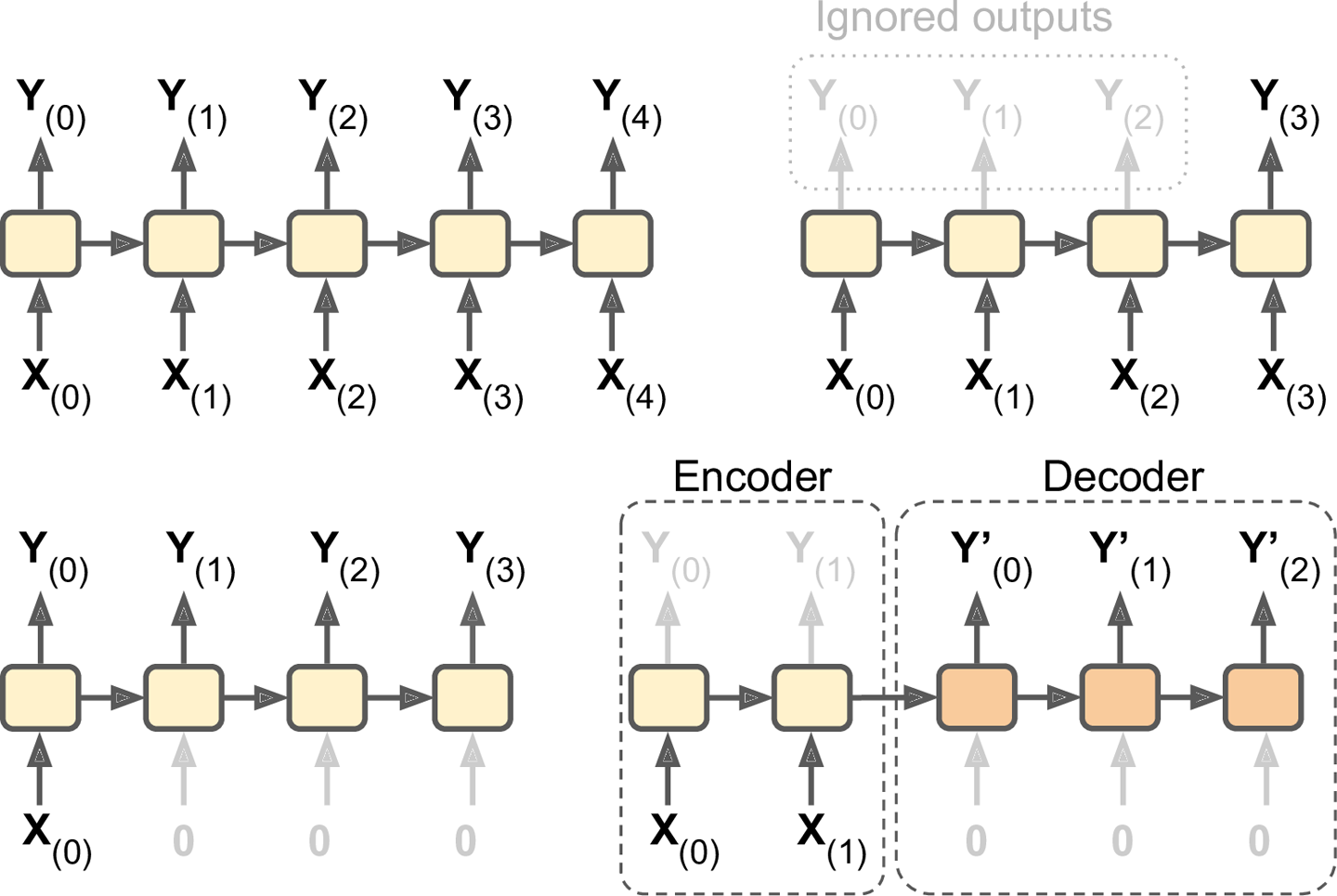## 14.2 基本RNN的TensorFlow实现

n_inputs = 3
n_neurons = 5

X0 = tf.placeholder(tf.float32, [None, n_inputs])
X1 = tf.placeholder(tf.float32, [None, n_inputs])

Wx = tf.Variable(tf.random_normal(shape=[n_inputs, n_neurons],dtype=tf.float32))
Wy = tf.Variable(tf.random_normal(shape=[n_neurons,n_neurons],dtype=tf.float32))
b = tf.Variable(tf.zeros([1, n_neurons], dtype=tf.float32))

Y0 = tf.tanh(tf.matmul(X0, Wx) + b)
Y1 = tf.tanh(tf.matmul(Y0, Wy) + tf.matmul(X1, Wx) + b)

init = tf.global_variables_initializer()


import numpy as np

# Mini-batch:         instance 0,instance 1,instance 2,instance 3
X0_batch = np.array([[0, 1, 2], [3, 4, 5], [6, 7, 8], [9, 0, 1]]) # t = 0
X1_batch = np.array([[9, 8, 7], [0, 0, 0], [6, 5, 4], [3, 2, 1]]) # t = 1

with tf.Session() as sess:
init.run()
Y0_val, Y1_val = sess.run([Y0, Y1], feed_dict={X0: X0_batch, X1: X1_batch})


>>> print(Y0_val) # output at t = 0
[[-0.2964572 0.82874775 -0.34216955 -0.75720584 0.19011548] # instance 0
[-0.12842922 0.99981797 0.84704727 -0.99570125 0.38665548] # instance 1
[ 0.04731077 0.99999976 0.99330056 -0.999933 0.55339795] # instance 2
[ 0.70323634 0.99309105 0.99909431 -0.85363263 0.7472108 ]] # instance 3
>>> print(Y1_val) # output at t = 1
[[ 0.51955646 1. 0.99999022 -0.99984968 -0.24616946] # instance 0
[-0.70553327 -0.11918639 0.48885304 0.08917919 -0.26579669] # instance 1
[-0.32477224 0.99996376 0.99933046 -0.99711186 0.10981458] # instance 2
[-0.43738723 0.91517633 0.97817528 -0.91763324 0.11047263]] # instance 3

### 14.2.1 随时间静态展开

static_rnn()函数可以创建一个展开的RNN。以下代码可创建与先前相同的模型：

X0 = tf.placeholder(tf.float32, [None, n_inputs])
X1 = tf.placeholder(tf.float32, [None, n_inputs])

basic_cell = tf.contrib.rnn.BasicRNNCell(num_units=n_neurons)
output_seqs, states = tf.contrib.rnn.static_rnn(basic_cell, [X0, X1], dtype=tf.float32)

Y0, Y1 = output_seqs


X = tf.placeholder(tf.float32, [None, n_steps, n_inputs])
X_seqs = tf.unstack(tf.transpose(X, perm=[1, 0, 2]))

basic_cell = tf.contrib.rnn.BasicRNNCell(num_units=n_neurons)
output_seqs, states = tf.contrib.rnn.static_rnn(basic_cell, X_seqs, dtype=tf.float32)

outputs = tf.transpose(tf.stack(output_seqs), perm=[1, 0, 2])


X_batch = np.array([
# t = 0      t = 1
[[0, 1, 2], [9, 8, 7]], # instance 0
[[3, 4, 5], [0, 0, 0]], # instance 1
[[6, 7, 8], [6, 5, 4]], # instance 2
[[9, 0, 1], [3, 2, 1]], # instance 3
])
with tf.Session() as sess:
init.run()
outputs_val = outputs.eval(feed_dict={X: X_batch})


### 14.2.2 随时间动态展开

dynamic_rnn()函数通过while_loop()对cell运算适当地次数。还可以设置swap_memory=True，在反向传播时交换GPU内存和CPU内存来防止OOM错误。更方便的是，它可以接受形状为[None, n_steps, n_inputs])的张量，这就不需要stack，unstack，以及transpose。下面简洁的代码实现了相同的模型：

X = tf.placeholder(tf.float32, [None, n_steps, n_inputs])

basic_cell = tf.contrib.rnn.BasicRNNCell(num_units=n_neurons)
outputs, states = tf.nn.dynamic_rnn(basic_cell, X, dtype=tf.float32)


### 14.2.3 处理变长输入序列

seq_length = tf.placeholder(tf.int32, [None])

[...]
outputs, states = tf.nn.dynamic_rnn(basic_cell, X, dtype=tf.float32, sequence_length=seq_length)


X_batch = np.array([
# step 0 step 1
[[0, 1, 2], [9, 8, 7]], # instance 0
[[3, 4, 5], [0, 0, 0]], # instance 1 (padded with a zero vector)
[[6, 7, 8], [6, 5, 4]], # instance 2
[[9, 0, 1], [3, 2, 1]], # instance 3
])
seq_length_batch = np.array([2, 1, 2, 2])

with tf.Session() as sess:
init.run()
outputs_val, states_val = sess.run([outputs, states], feed_dict={X: X_batch, seq_length: seq_length_batch})


## 14.3 训练模型### 14.3.1 训练一个序列分类器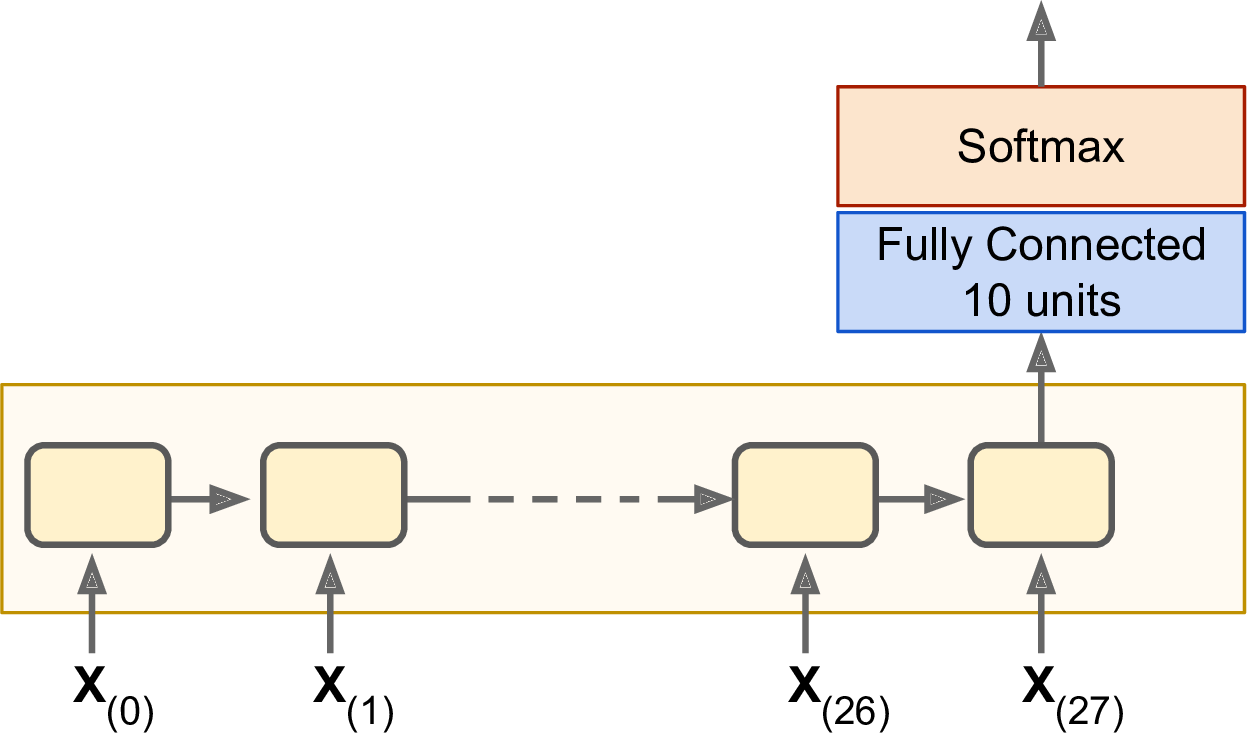from tensorflow.contrib.layers import fully_connected

n_steps = 28
n_inputs = 28
n_neurons = 150
n_outputs = 10

learning_rate = 0.001

X = tf.placeholder(tf.float32, [None, n_steps, n_inputs])
y = tf.placeholder(tf.int32, [None])

basic_cell = tf.contrib.rnn.BasicRNNCell(num_units=n_neurons)
outputs, states = tf.nn.dynamic_rnn(basic_cell, X, dtype=tf.float32)

logits = fully_connected(states, n_outputs, activation_fn=None)
xentropy = tf.nn.sparse_softmax_cross_entropy_with_logits(labels=y, logits=logits)

loss = tf.reduce_mean(xentropy)
optimizer = tf.train.AdamOptimizer(learning_rate=learning_rate)
training_op = optimizer.minimize(loss)
correct = tf.nn.in_top_k(logits, y, 1)
accuracy = tf.reduce_mean(tf.cast(correct, tf.float32))

init = tf.global_variables_initializer()


from tensorflow.examples.tutorials.mnist import input_data

mnist = input_data.read_data_sets("/tmp/data/")
X_test = mnist.test.images.reshape((-1, n_steps, n_inputs))
y_test = mnist.test.labels


n_epochs = 100
batch_size = 150

with tf.Session() as sess:
init.run()
for epoch in range(n_epochs):
for iteration in range(mnist.train.num_examples // batch_size):
X_batch, y_batch = mnist.train.next_batch(batch_size)
X_batch = X_batch.reshape((-1, n_steps, n_inputs))
sess.run(training_op, feed_dict={X: X_batch, y: y_batch})
acc_train = accuracy.eval(feed_dict={X: X_batch, y: y_batch})
acc_test = accuracy.eval(feed_dict={X: X_test, y: y_test})
print(epoch, "Train accuracy:", acc_train, "Test accuracy:", acc_test)


### 14.3.2 训练时序数据

这次我们处理时序数据，比如股价、气温、脑电波等等。每一个训练实例都是从时序中随机选出20个时刻（如图14-7左侧）。目标序列与训练序列是相同的，只不是目标序列始终比训练序列晚一个时刻（如图14-7右侧，最下角的实心篮圈，是$t_0$时刻的输入。紧接着的空心篮圈，是$t_1$时刻的输入同时也是$t_0$时刻的目标值。依此类推）。n_steps = 20
n_inputs = 1
n_neurons = 100
n_outputs = 1

X = tf.placeholder(tf.float32, [None, n_steps, n_inputs])
y = tf.placeholder(tf.float32, [None, n_steps, n_outputs])
cell = tf.contrib.rnn.BasicRNNCell(num_units=n_neurons, activation=tf.nn.relu)
outputs, states = tf.nn.dynamic_rnn(cell, X, dtype=tf.float32)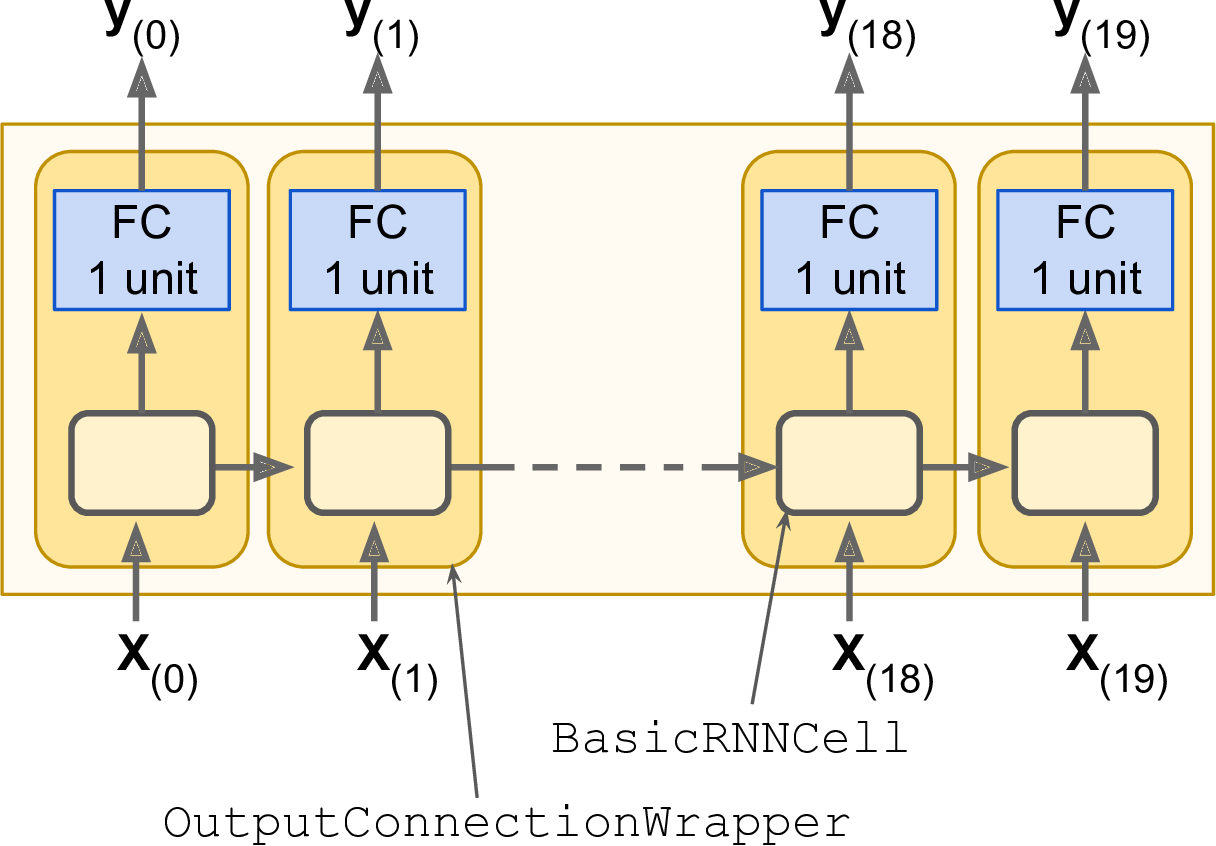cell = tf.contrib.rnn.OutputProjectionWrapper(
tf.contrib.rnn.BasicRNNCell(num_units=n_neurons, activation=tf.nn.relu),
output_size=n_outputs)


learning_rate = 0.001

loss = tf.reduce_mean(tf.square(outputs - y))
optimizer = tf.train.AdamOptimizer(learning_rate=learning_rate)
training_op = optimizer.minimize(loss)

init = tf.global_variables_initializer()


n_iterations = 10000
batch_size = 50

with tf.Session() as sess:
init.run()
for iteration in range(n_iterations):
X_batch, y_batch = [...] # fetch the next training batch
sess.run(training_op, feed_dict={X: X_batch, y: y_batch})
if iteration % 100 == 0:
mse = loss.eval(feed_dict={X: X_batch, y: y_batch})
print(iteration, "\tMSE:", mse)


0   MSE: 379.586
100 MSE: 14.58426
200 MSE: 7.14066
300 MSE: 3.98528
400 MSE: 2.00254
[...]


X_new = [...] # New sequences
y_pred = sess.run(outputs, feed_dict={X: X_new})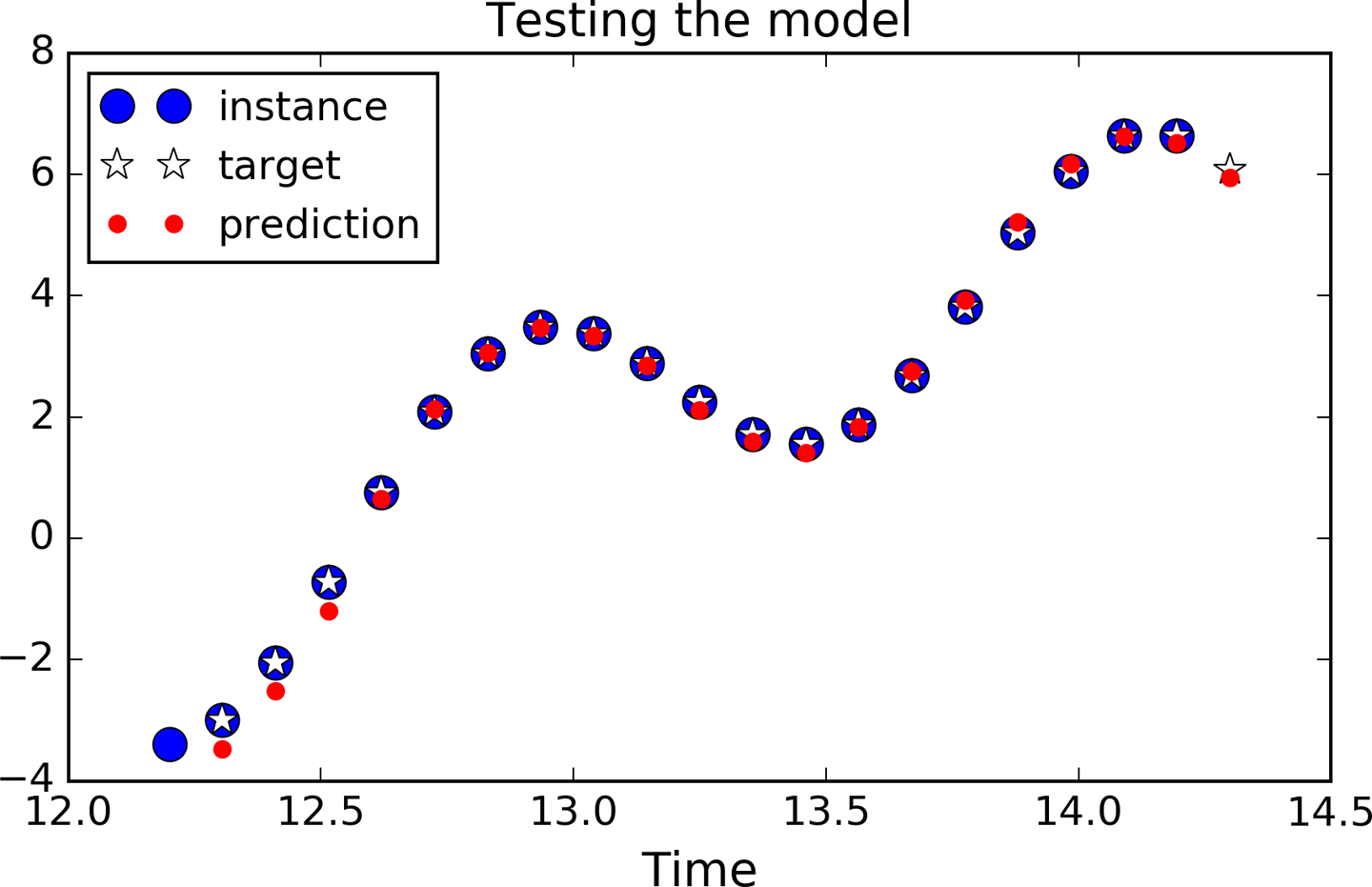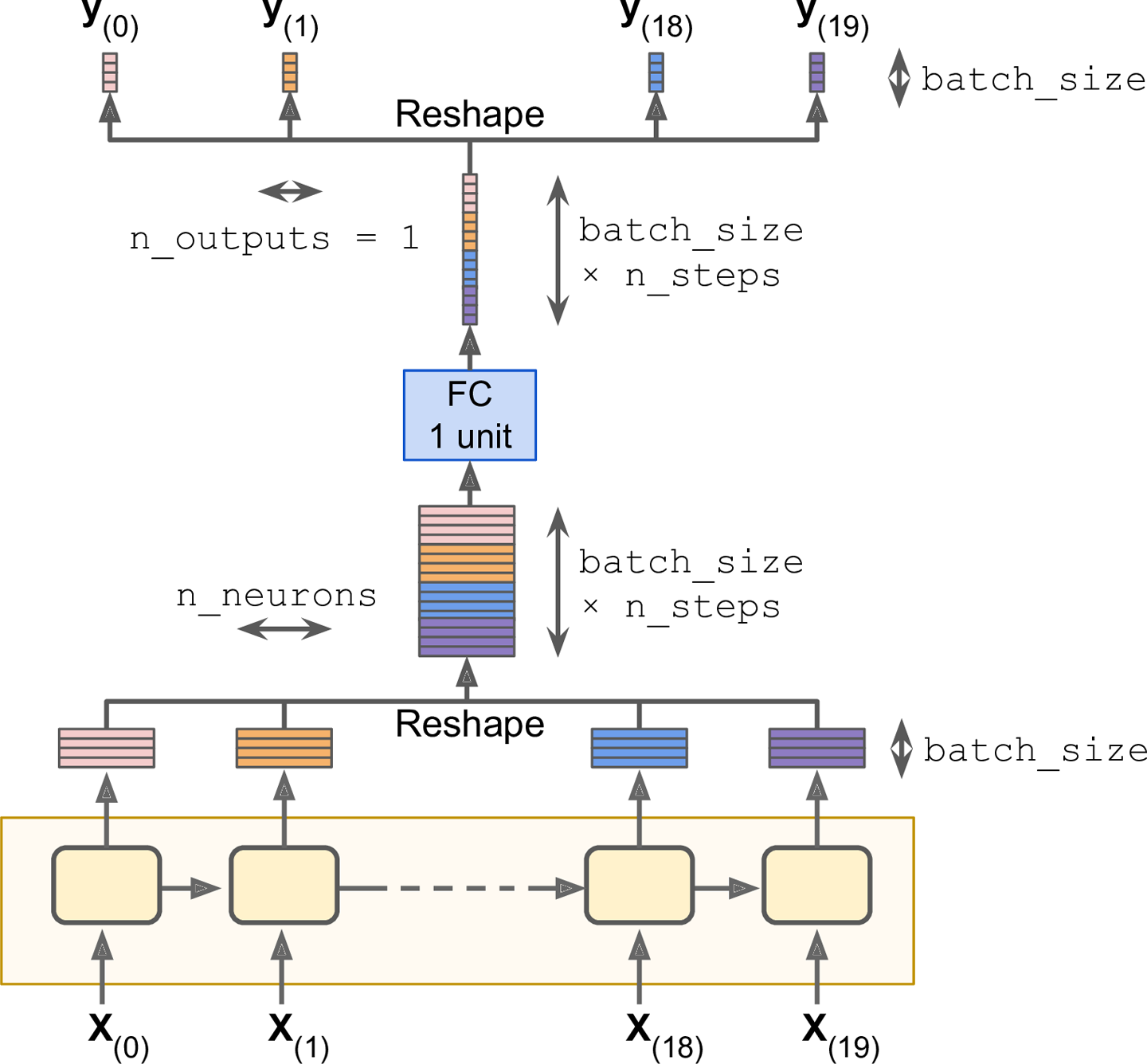cell = tf.contrib.rnn.BasicRNNCell(num_units=n_neurons, activation=tf.nn.relu)
rnn_outputs, states = tf.nn.dynamic_rnn(cell, X, dtype=tf.float32)


stacked_rnn_outputs = tf.reshape(rnn_outputs, [-1, n_neurons])
stacked_outputs = fully_connected(stacked_rnn_outputs, n_outputs, activation_fn=None)
outputs = tf.reshape(stacked_outputs, [-1, n_steps, n_outputs])


### 14.3.3 创造性的RNN

sequence = [0.] * n_steps
for iteration in range(300):
X_batch = np.array(sequence[-n_steps:]).reshape(1, n_steps, 1)
y_pred = sess.run(outputs, feed_dict={X: X_batch})
sequence.append(y_pred[0, -1, 0])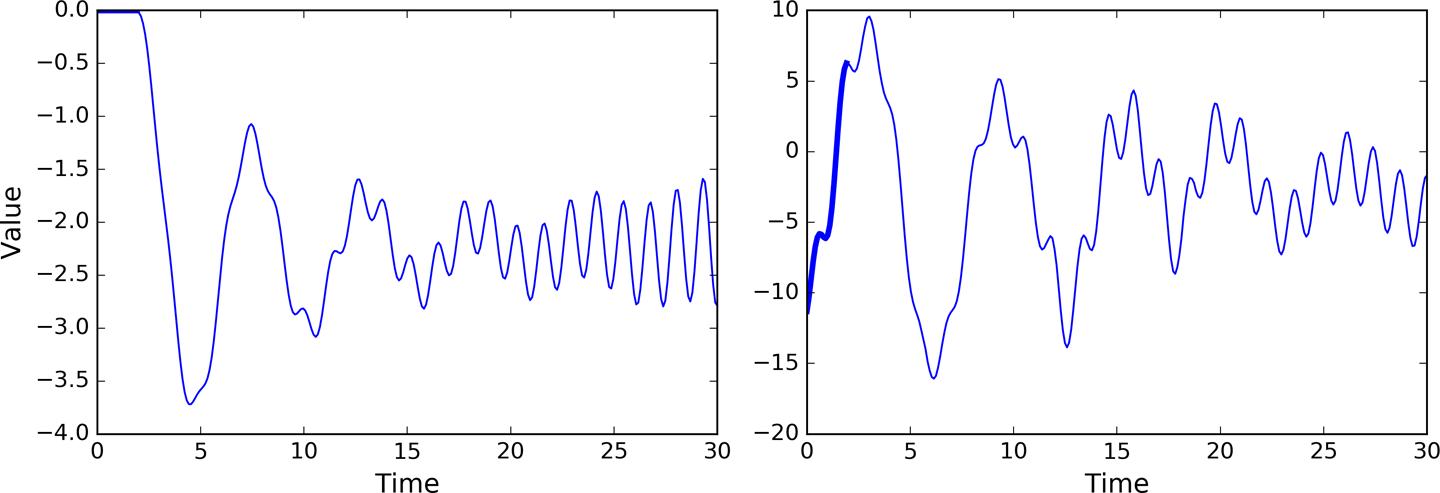posted on 2018-05-25 15:55  royhoo  阅读(20209)  评论(0编辑  收藏  举报